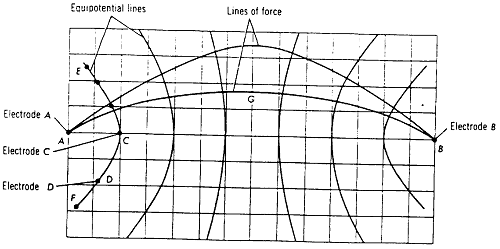# Lab: Electric Field Lines

## Mapping an Electric Field – Demo Lab

Problem:How can an electric field be mapped?
An electric field exists around every charged object. If a unit positive charge is placed in this field, a force is exerted on the unit charge by the field. The direction of this force is the direction of the field, and its magnitude is the strength of the field. In this experiment you are going to learn how to make a map of the electric field between oppositely charged objects.

Needed Information and Skills:
A difference of potential exists between two points in an electric field if work is required to move a unit positive charge from one point to the other against the force exerted on the unit charge by the field. If no work is done in moving a unit charge from one point to another, the points are said to be at the same potential. Lines in an electric field joining all such points are called equipotential lines.
In this experiment you are going to map the equipotential lines of an electric field between two electrodes immersed in a conductive water solution as shown in Fig. 1. The field will be set up by applying an AC voltage to these electrodes instead of a DC voltage. Since each electrode will become alternately positively and negatively charged, the electric field between these electrodes will continually change direction. However, the equipotential lines between the electrodes will remain constant in position and may therefore be located.
To map the equipotential lines, there will be second pair of electrodes which are connected to speaker. When these two probing electrodes are put in the conductive water at any two points which are at different potentials, alternating current will flow in the earphones and produce a tone. If one of the probing electrodes is then kept fixed and the position of the second electrode is changed, a series of points can be found for which the tone in the earphones decreases to zero. All such "zero-tone" points located by the second probe are at the same potential as the fixed electrode and a line drawn through these points is an equipotential line.
The lines of force of an electric field are perpendicular to the equipotential lines. Hence, once you have drawn the equipotential lines of an electric field, you can draw the lines of force of the field by drawing continuous lines perpendicular to the suc-cessive equipotential lines as shown in Fig. 2.

Gathering the Data:
The instructor will perform the experiment.  You will need to record on your graph paper all equipotential points as they are discovered.

Solving the Problem:
On the coordinate paper on which you have mapped equipotential lines between electrodes A and B draw a line such as AGB which always intersects the successive equipotential lines at right angles. See Fig. 2. This line represents a line of force. In a similar fashion draw additional lines between A and B until you have mapped the field between A and B with about 6 to 10 lines of force.Using the above procedure, set up an electric field between two parallel straight metal electrodes about 14 cm apart in the conductive solution by connecting A and B to the electrodes. Map several equipotential lines between these electrodes. From the equipotential pattern, draw 6 to 8 lines of force between the electrodes.
If time permits, map the lines of force between an differently shaped electrodes.